# High School Geometry - Chords, Secants and Tangents

## Introduction

• A chord is a line segment joining any two points on the boundary of a circle.
• A line intersecting a circle at two distinct points is called a Secant.
• A line intersecting or touching a circle at only one point is called its tangent.

## Important Points

• The measure of an angle formed by two chords intersecting inside a circle is equal to half the sum of the measure of the arcs intercepted by the angle and its vertical angle counterpart.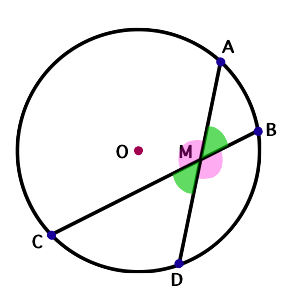In the figure shown above, $m\angle AMB=m\angle CMD=\frac{1}{2}\left(m\stackrel{⏜}{CD}+m\stackrel{⏜}{AB}\right)$

• The measure of an angle formed by a tangent and a chord meeting at the point of tangency is half the measure of the intercepted arc.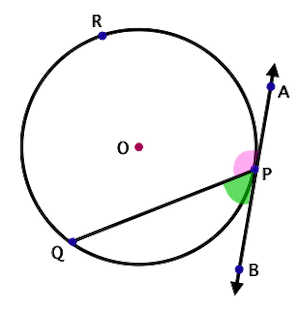In the figure shown above, $m\angle BPQ=\frac{1}{2}m\stackrel{⏜}{QP}$ and $m\angle APQ=\frac{1}{2}m\stackrel{⏜}{QRP}$

• If two secants intersect outside a circle, then the measure of the angle formed is equal to half the positive difference of the measures of the intercepted arcs.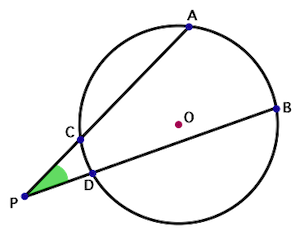In the figure shown above, $m\angle APB=\frac{1}{2}\left|m\stackrel{⏜}{AB}-m\stackrel{⏜}{CD}\right|$

• If the two chords of a circle are equal in measure, then their corresponding minor arcs are equal in measure, and vice-versa.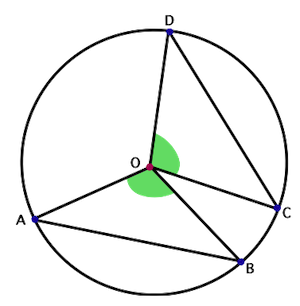In the figure above, chords AB and CD are equal in measure. Thus, $m\stackrel{⏜}{AB}=m\stackrel{⏜}{CD}$.

• If the diameter of a circle is perpendicular to a chord, then it bisects the chord and its arcs.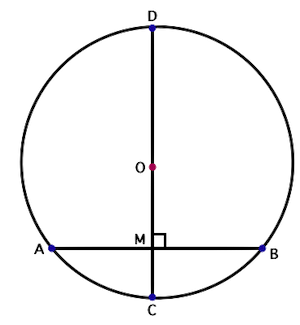In the figure shown above, if the diameter CD is perpendicular to the chord AB, then AM = BM.

• If two chords of a circle are equal in measure, then they are equidistant from the center.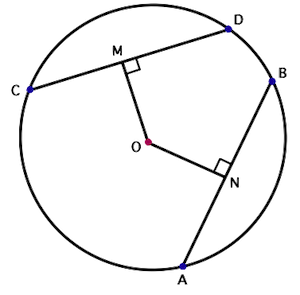In the figure shown above, if chords AB and CD are equal, then OM = ON.

• If two chords intersect inside a circle, then the product of the segments of one chord equals the product of the segments of the other chord.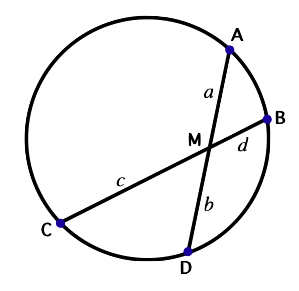In the figure shown above, $a×b=c×d$

• If two secant segments intersect outside a circle, then the product of the secant segment with its external portion equals the product of the other secant segment with its external portion.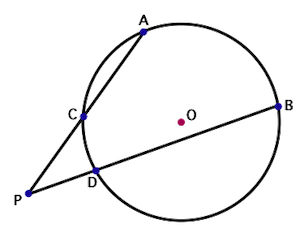In the figure shown above, $PA×PC=PB×PD$

• If a tangent segment and a secant segment intersect outside a circle, then the square of the measure of the tangent segment equals the product of the measures of the secant segment and its external portion.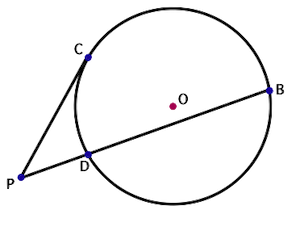In the figure shown above, ${\left(PC\right)}^{2}=PB×PD$

• The lengths of tangents drawn from an external point to a circle are equal.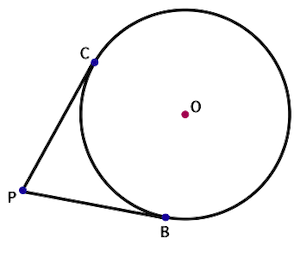In the figure shown above, $PB=PC$

## Solved Examples

Example 1: In the figure shown $m\stackrel{⏜}{AB}=120°$ and $m\stackrel{⏜}{CD}=100°$. Find the measure of $x$.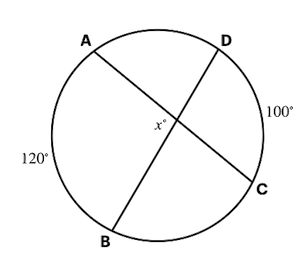Solution: $x=\frac{1}{2}×\left(120°+100°\right)$ $=\frac{1}{2}×220°$ $=110°$

Example 2:  Find the measure of $\angle BPQ$ as shown in the figure if $m\stackrel{⏜}{PQ}=108°$.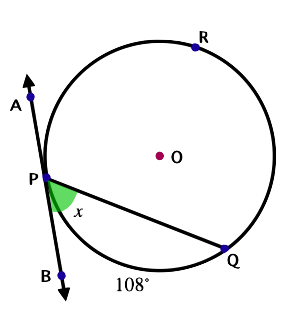Solution: $\angle BPQ=\frac{1}{2}m\stackrel{⏜}{PQ}$ $=\frac{1}{2}×108°$ $=54°$

Example 3: In the figure shown, $m\stackrel{⏜}{CD}=16°$ and $m\stackrel{⏜}{AB}=58°$. Find the measure of $\angle APB$.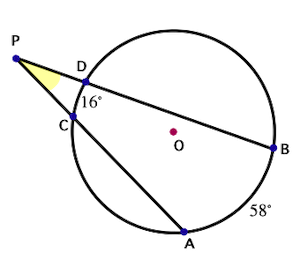Solution: $\angle APB=\frac{1}{2}\left|m\stackrel{⏜}{AB}-m\stackrel{⏜}{CD}\right|$ $=\frac{1}{2}\left|58°-16°\right|$ $=\frac{1}{2}×42°$ $=21°$

Example 4: Find the measure of the unknown length $x$ shown in the figure.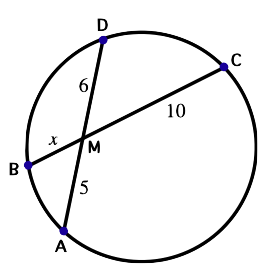Solution: $10x=6×5$

$x=\frac{6×5}{10}$

Example 5: In the figure shown, if  and , find the length of CD.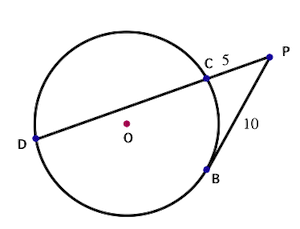Solution: ${\left(PB\right)}^{2}=PC×PD$

${\left(10\right)}^{2}=5×PD$

$PD=PC+CD$

$20=5+CD$

## Cheat Sheet

• The measure of an angle formed by two secants, by two tangents, or by a secant and tangent intersecting in the exterior of a circle is one-half the difference between the measures of the intercepted arcs.
• If two chords intersect inside a circle, then the product of the segments of one chord equals the product of the segments of the other chord.
• If two secant segments intersect outside a circle, then the product of the secant segment with its external portion equals the product of the other secant segment with its external portion.
• If a tangent segment and a secant segment intersect outside a circle, then the square of the measure of the tangent segment equals the product of the measures of the secant segment and its external portion.
• The tangent at any point of a circle is perpendicular to the radius through the point of contact.
• The lengths of tangents drawn from an external point to a circle are equal.

## Blunder Areas

• Tangent to a circle is a special secant in which the two endpoints of its corresponding chord coincide.